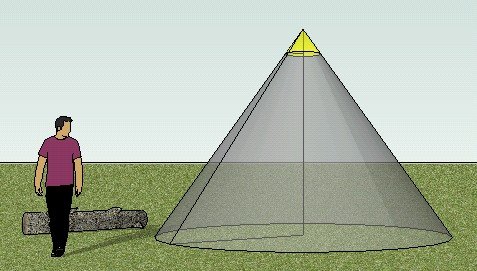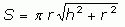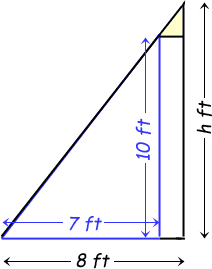SEARCH HOMEMath Central Quandaries & QueriesQuestion from Lorne, a parent: What is the surface area of an open ended cone? Measured at 10' high, 16' diameter on the bottom and 2' diameter at the top.Hi Lorne.

The surface area of the main "open-ended" cone is the same as the surface area of a full (closed) cone minus the surface area of the cone that is a cap on the top.

The surface area S of a cone is calculated based on the height h and the radius r at the base:So we need to find these quantities first.Notice that the cross-section shown here reveals the similar triangles involved in the geometry.

In similar triangles, the ratios of the side lengths is the same. This means that 10/7 = h/8. Thus, the h = 80/7 ft.

If we use the formula for surface area above with r = 8 and h = 80/7, we can calculate the surface area of the full large (closed) cone. The surface area of the cap would come from using r = 1 and h = 80/7 - 10.

Subtract these surface areas to complete the problem.

Cheers,
Stephen La Rocque.Math Central is supported by the University of Regina and The Pacific Institute for the Mathematical Sciences.